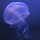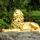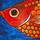## General Question# How can two infinite sets not have one to one correspondence with each other?

Asked by jmilk (7) 2 weeks ago

Recently I watched Veritasium’s video on math’s fatal flaw. I understood some of it but one part that I particularly struggled with was Cantor’s diagonal argument. I did some research on bijection and I understand what constitutes a one to one correspondence between two sets, however what I don’t understand is how two infinite sets could possibly ever not have a one to one correspondence. Because from my limited understanding of mathematics, infinity seems to be a characteristic of a set that means its cardinality does not exist, because their is no bounds or ends to a set. I understand how the new real number is generated in Cantor’s proof, but I don’t understand how when matching the sets that one set of boundless numbers could be any more or less boundless then another. Put more colloquially, because I’m bad at phrasing this question in advanced mathematical terminology, no matter how many numbers are created using the diagonal proof, is there not another natural number that can always be matched up against it, given that the amount of natural numbers is boundless?

Observing members: 0Composing members: 0## 4 AnswersYou’ve missed what an “infinite set” means.

Consider this definition: “Infinite sets are the sets containing an uncountable or infinite number of elements. Infinite sets are also called uncountable sets.”

That is, calling a set infinite, simple means it includes an uncountably large number of members. If you list X members, someone could always mention more members.

So these are all infinite sets:

* All the real numbers between 1 and 2.
* All the real numbers between 3 and 4.
* All the points on the line x = y.
* All the points on the line x = y + 3.

There an an infinite number of members of the first set and the second set, and none of the members of the first set are in the second set.

Same for the third and fourth sets.

Or for “the set of all positive integers” and “the set of all negative integers”.

Zaku (26398)“Great Answer” (3) Flag as…(That definition was from this site: https://www.storyofmathematics.com/infinite-sets which has some other nice explanations.)

Zaku (26398)“Great Answer” (1) Flag as…It does seem counter-intuitive, but the proof is quite clear. Given any one-to-one correspondence from rationals to reals, the diagonal process provides a way of generating infinitely many real numbers that are not included.

How can you argue against this proof: Since the rationals are countable, we can match them to the positive integers. We can now think of each integer matching to a real number. To find a real number not on the list, choose a number whose first digit is different from the first digit of the real number matched to 1, and whose second digit is different from the second digit of the number matched to 2, and so on. We end up with a number not on the list, and we could find infinitely many such numbers. That means a one-to-one correspondence is impossible.

The trick is the use of one-to-one correspondence as a means for comparing two infinities. Do you have a better method?

LostInParadise (28692)“Great Answer” (1) Flag as…You can make a start counting integers, 1. 2. 3. 4……… it’s just that you have to go on and on forever but how do you count the number of points in a line? The first point can be called 1 but you immediately get into trouble as how do you name the second point? It would take an infinite number of decimals to define this point and so you have to give up and say it is uncountable and there is no one to one correspondence with the integers.

flutherother (31064)“Great Answer” (1) Flag as…## Answer this questionor

#### Join

to answer.

This question is in the General Section. Responses must be helpful and on-topic.

Your answer will be saved while you login or join.
Have a question? Ask Fluther!
What do you know more about?
or
Knowledge Networking @ Fluther